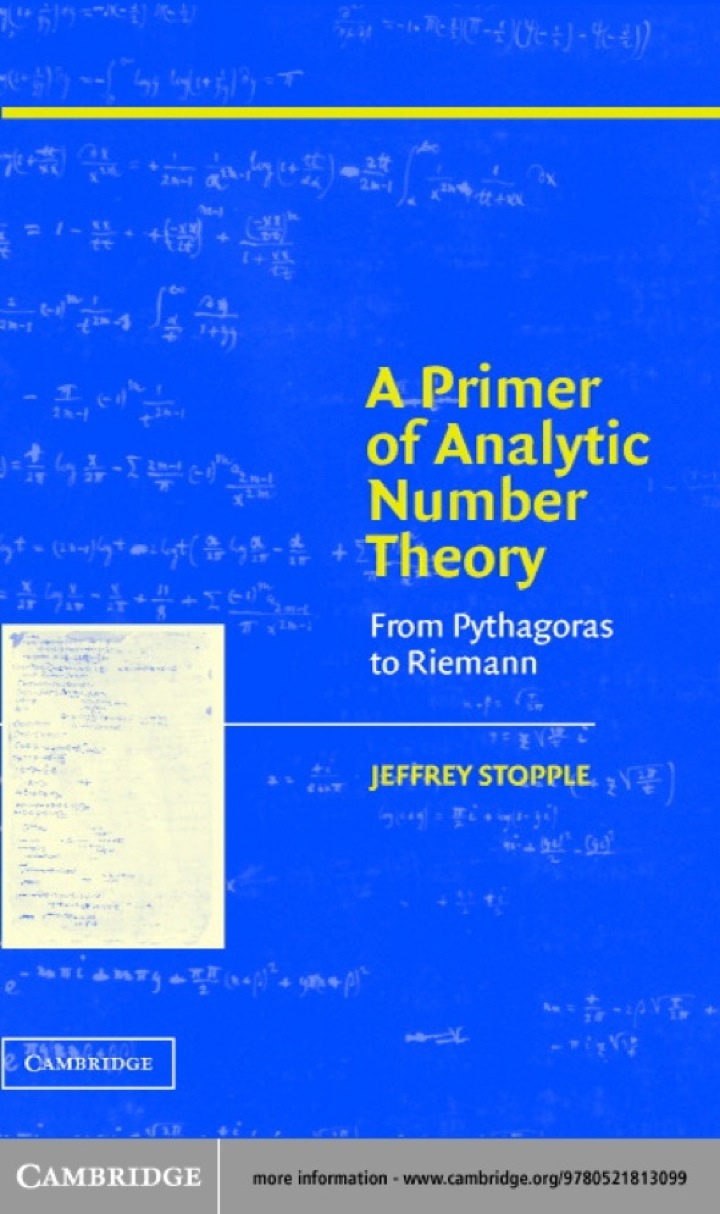# A Primer of Analytic Number Theory

## A Primer of Analytic Number Theory

By Jeffrey Stopple

Expires on May 8th, 2022
\$53

Publisher List Price: \$0.00

This 2003 undergraduate introduction to analytic number theory develops analytic skills in the course of studying ancient questions on polygonal numbers, perfect numbers and amicable pairs. The question of how the primes are distributed amongst all the integers is central in analytic number theory. This distribution is determined by the Riemann zeta function, and Riemann's work shows how it is connected to the zeroes of his function, and the significance of the Riemann Hypothesis. Starting from a traditional calculus course and assuming no complex analysis, the author develops the basic ideas of elementary number theory. The text is supplemented by series of exercises to further develop the concepts, and includes brief sketches of more advanced ideas, to present contemporary research problems at a level suitable for undergraduates. In addition to proofs, both rigorous and heuristic, the book includes extensive graphics and tables to make analytic concepts as concrete as possible.

Subject: Mathematics & Statistics -> Post-Calculus -> Number TheoryFrom Pythagoras to Riemann
1st edition
Publisher: Cambridge University Press 6/23/03
Imprint: Cambridge University Press
Language: English

ISBN 10: 0511058373
ISBN 13: 9780511058370
Print ISBN: 9780521813099

Live Chats